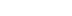/
article

# Using NURBS Surfaces in Real-Time Applications

The quest goes on to find suitable alternatives to polygonal models, and one of the most promising candidates seems to be NURBS surfaces. In this article, Dean Macri covers everything from concepts and terminology associated with parametric curves and surfaces, to difficulties encountered when using NURBS surfaces in place of polygonal models, to the types of 3D content that can be created by using parametrics.

Alternatives to Polygonal Models

Despite the widespread use of polygonal models for representing 3D geometry, the quest goes on to find suitable alternatives, particularly since the limitations of polygonal data have become glaringly obvious to current-generation developers. Because PC developers need to create content that scales across many levels of processor performance (including both host processors and 3D graphics accelerators), they're forced to either create multiple models or to use mesh reduction algorithms for dynamically producing the lower detail models. Creating multiple models clearly taxes the efforts of 3D artists, who must spend even more time modeling, manipulating, and animating models composed of large numbers of polygons. As games become more content intensive (not just in terms of the levels of detail, but more actual game content), the time required to produce the content grows considerably. Alternatives to polygonal models offer artists an acceptable means to streamline the creation process and save time along the way.

This article deals with one of the more promising alternatives to polygonal modeling: NURBS (Non-Uniform Rational B-Spline) surfaces. First, I'll introduce you to the concepts and terminology associated with parametric curves and surfaces. Next, I'll describe in detail how to render NURBS surfaces and discuss some of the difficulties encountered when using NURBS surfaces in place of polygonal models. Finally, if I've done my job well, this article will whet your appetite for the exciting types of 3D content that can be created using parametric surfaces and inspire you to investigate developing this type of content.

Parametric Curve Basics

Let's start with the basics. Normal "functions," as presented in algebra or calculus (or whatever mathematics course we've taken recently or not so recently) are defined as the dependent variable (often y) given as a function of the independent variable (usually x) so that we have an equation such as: y = 2x^2 - 2x + 1. By plugging in various values for x we can calculate corresponding values for y. We can create a graph of the function by plotting the corresponding x and y values on a two-dimensional grid, as shown in Figure 1.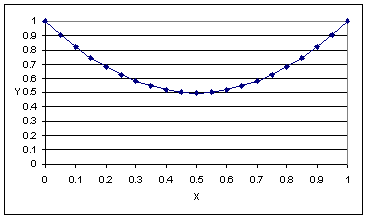Figure 1. An ordinary function.

Parametric functions also match values of x with values of y, but the difference is that both x and y are given as functions of a third variable (often represented by u) called the parameter. So we could have a set of equations expressed as follows:

y = 2u^2 - 2u +1
x = u

These equations produce the same curve that the "implicit" function given above produces. An additional restriction often added to parametric functions is that the functions are only defined for a given set of values of the parameter. In our simple example, u could be any real number but for many sets of equations, the equations will only be considered valid on a range such as 0 <= u <= 1.

Once you understand the nature of a parametric function, we can examine how this pertains to parametric curves and surfaces. In simplest terms, a parametric curve is the plot of a set of parametric functions over the valid parameter range. Our previous example has two functions (one for x and one for y) that when plotted for 0 <= u <= 1 create the graph in Figure 1. We can easily add a third function for z (such as: z = 2u) and then we have a set of parametric functions that create a curve in 3-space.

That's all well and good, you might be thinking, but how do these parametric functions get chosen in a way that is useful to software developers? Essentially, typical "parametric" curves and surfaces are more than just a set (or sets) of parametric functions. Let's take the earlier description one step further so that we can see how parametric curves and surfaces originate.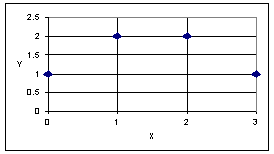Figure 2. A set of 2-dimensional points.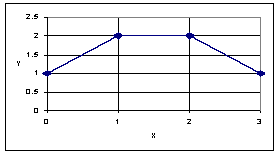Figure 3. The same set of points, connected linearly.

Consider the set of 2-dimensional points in Figure 2 as well as the linear connection of these points shown in Figure 3. We can think of these points as representing a linear approximation of a curve that starts at the first point (0,1) and ends at the last point (3,1). Another way of looking at this: as x goes from 0 to 1, y goes from 1 to 2. As x goes from 1 to 2, y stays at 2, and as x goes from 2 to 3, y goes from 2 down to 1. In mathematical terms, the "curve" in Figure 3 can be defined as a "blending" of four points: P0 = (0,1), P1 = (1,2), P2 = (2,2), and P3 = (3,1). The points are blended by a set of functions defined as follows:

 F0(u) = u if 0 <= u < 1 = 0 otherwise F1(u) = 1-u if 0 <= u < 1 = 1 if 1 <= u < 2 = 0 otherwise F2(u) = 1 if 1 <= u < 2 = u-2 if 2 <= u £ 3 = 0 otherwise F3(u) = u-2 if 2 <= u £ 3 = 0 otherwise

Now, the curve (we'll call it C) can be defined as:

C(u) = F0(u)*P0 + F1(u)*P1 + F2(u)*P2 + F3(u)*P3

This gives us a two-dimensional curve (C) defined as a linear combination of four two-dimensional points (P0,P1,P2,P3) and four scalar parametric blending functions (F0,F1,F2,F3) valid in the closed interval [0,3].

Are you excited, yet? Probably not, but I am because here's the kicker: by creatively choosing these blending functions, we can change the look and smoothness of the curve both in terms of visual appearance and mathematical continuity.

You may still be wondering how we'll come up with these blending functions more easily. Well, part of the secret lies in the concept of a knot vector. A knot vector is simply a vector (which is a list of one or more real numbers) that describes the "knots" of the curve. Think of a knot as a point where the blending functions change. In the previous example, the blending functions change at 0 (where they start), 1, and 2. This is apparent from the conditions on the functions (such as 1 <= u < 2). An example of a knot vector would be: {0, 1, 2, 3}. We'll call this knot vector U and denote each of the terms in it as u0,u1,u2,u3 so that u0=0, u1=1, u2=2, and u3=3.

Knot vectors have the following characteristics:

1. The values must be non-decreasing. This means that ui+1 >= ui for all i. This also means that values can be repeated so that {0,1,1,2,3} is a valid knot vector.
2. The spacing of the "knots" (that is, the difference between successive knot values ui and ui+1) can either be "uniform" (the same for all uI and ui+1 pairs) or "non-uniform". We'll talk about this later in the article.
3. The number of elements, m, in a knot vector must be defined by m = p + n + 1 where n is the number of control points and p is the degree of the desired blending function (as shown in a later example).

B-Spline Basis Functions

Now we're going to define a powerful set of parametric functions called the b-spline basis functions (the b in b-spline stands for "basis" so this term is kind of redundant). These equations are defined for a given knot vector U = {u0, u1, …, un} as given in Equation 1.

Equation 1: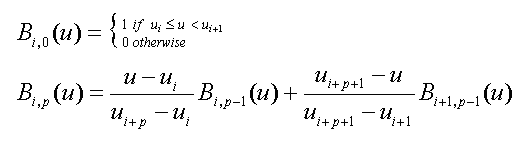Whoa, that's scary! Let's take a close look at it to see what makes it useful. The p subscript in the second equation is the degree of the function (points are zero'th degree, lines are first degree, and so on). The first equation expresses that for zero'th degree curves, the function is either constant zero or constant one depending on the parameter, u, and where it falls in the knot vector. Looking at this pictorially for the knot vector U = {0,1,2} and B0,0, B1,0, and B2,0 we get the plots shown in Figure 4.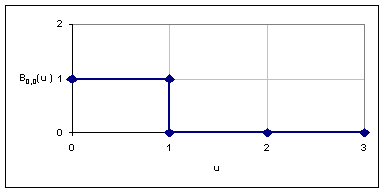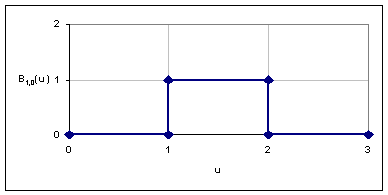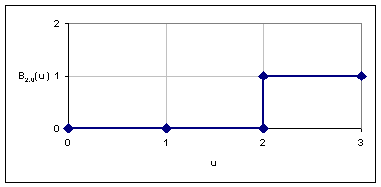Figure 4. Plots of U = {0,1,2} for B0,0, B1,0, and B2,0

For degrees other than zero, we must recursively calculate the value of the function using a linear combination of the functions that are one degree less than the degree for which we're calculating. For first degree functions, we use a linear combination of the zero'th degree functions. For second degree functions, we use a linear combination of the first degree functions (which are also defined as a linear combination of the zero'th degree functions), and so on. As an example, for the knot vector U = {0,1,2, 3} we produce the plots shown in Figure 5 for B0,1, B1,1, B2,1, and B3,1.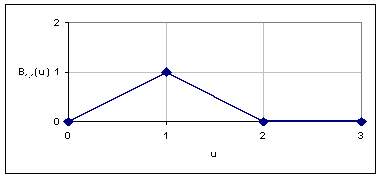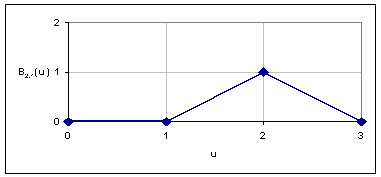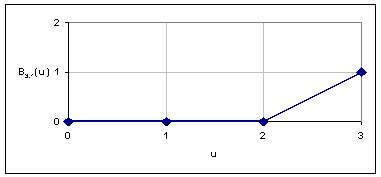Figure 5. Plots of U = {0,1,2} for B0,1, B1,1, B2,1, and B3,1.1

Interestingly enough, with the four control points, P0, P1, P2, and P3 defined in our previous example, we can now represent the curve, C from Figure 3, as a parametric curve by the equation:

C(u) = B0,1(u) * P0 + B1,1(u) * P1 + B2,1(u) * P2 + B3,1(u) * P3 with knot vector U = {0,1,2,3}.

This can be expressed more compactly as: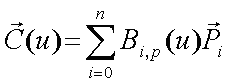In our example, n = 3 and p = 1.

To verify that this approach works, pick a value for u, say 1.5. Looking at the plots in Figure 5 we can see that:

B0,1(1.5) = 0
B1,1(1.5) = 0.5
B2,1(1.5) = 0.5
B3,1(1.5) = 0

Looking at just the x values of the points, we get:

 CX(1.5) = B0,1(1.5) x P0,X + B1,1(1.5) x P1,X + B2,1(1.5) x P2,X + B3,1(1.5) x P3,X = 0 * 0 + 0.5*1 + 0.5*2 + 0*0 = 1.5

Looking at the y values of the points, we get :

 CY(1.5) = B0,1(1.5) * P0,Y + B1,1(1.5) * P1,Y + B2,1(1.5) * P2,Y + B3,1(1.5) * P3,Y = 0 * 0 + 0.5*2 + 0.5*2 + 0*0 = 2

Therefore, C(1.5) = (1.5, 2) which is just what we expect it to be!

We've covered a lot of ground and still haven't even looked at parametric surfaces yet. That's okay because by now you should have a decent understanding of the nature of parametric surfaces. We know that a parametric function is a set of equations that produce one or more values for a given parameter. In our examples, we produced x and y values and could easily have produce z values to generate points in 2-space or 3-space. I've also shown how several parametric functions can be used to "blend" points in 2-space (again, blending in 3-space would be a trivial extension of this process). We also learned what a knot vector is and how knot vectors can be used together with the b-spline basis functions to create some interesting "blending" functions.

Parametric Surfaces

Now that we know how to describe parametric curves using a set of control points (which is what P0, P1, P2, and P3 were in the previous example), we can begin to understand parametric surfaces. The control points that we're going to use for parametric surfaces will be 3-dimensional points. Let's construct an example using the points shown in Figure 6.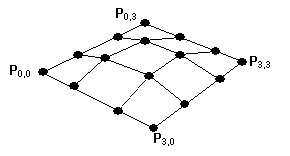Figure 6. A parametric surface.

Starting with16 points labeled P0,0 through P3,3, we want to "blend" these points together to form a surface. This process is actually quite easy. To generate a surface point that we'll call S, start with two knot vectors, U and V, to create two sets of b-spline basis functions, Bi,p(u) and Bj,q(v). Here p and q tell us the degrees of the surface (for example: linear, quadratic, cubic) in each direction. Now, we can define the function for the surface that corresponds to the function for a curve shown in Equation 3: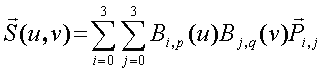Simple enough? Let's look at it in greater depth just to be sure that the process is clear. To calculate a surface point, S(u,v), we loop over all the control points (with the two summation signs in the equation) and scale each control point, Pi,j, by the appropriate blending functions evaluated at u and v. Keep in mind that for a surface with many control points, some of the blending functions will be equal to zero over large regions of the surface. In particular, for a surface of degree n x m, at most (n+1)*(m+1) blending functions will be non-zero at a given (u,v) parameter value.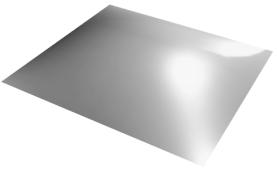Figure 7. The generated surface.

We can generate different surfaces by using different knot vectors and changing the degrees of the blending functions (p and q). For example, if you generate a surface that is 3rd degree in both dimensions with knot vectors U = {0,0,0,0,1,1,1,1} and V = {0,0,0,0,1,1,1,1], the result would look like the image in Figure 7 if we use the control point mesh from Figure 6.

If you're wondering why we chose these particular knot vectors, the reason is simple. By having the repeated knot values at the beginning and end of the vectors, the resulting surface interpolates (in other words it passes through) the corner and edge control points. In contrast, the surface drawn does not pass through the middle control points, although it does approach them.

Before getting to the sample code, let's cover one more thing. The basis functions that we've described have an interesting property (actually it's by design). If you expand them for a given degree, n, and a fixed knot vector, you end up with a polynomial equation of the form: A0 + A1u + A2u^2 + A3u^3 + … + Anu^n where AI are coefficients that are determined exclusively by the knot vector and degree. Polynomials are good functions used for approximating (or, in some cases, representing exactly) other functions. However, there are some three dimensional surfaces that can't easily be approximated using polynomials as bases; specifically, the conics: spheres, cylinders, cones, and so on. To more easily and accurately represent these surfaces, you can use a ratio of polynomials. For two polynomial equations, F and G, a rational polynomial R would be defined by: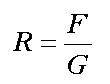Using the b-spline functions from Equation 1, we can define a "rational" parametric surface by adding to the control points a fourth component (the first three are x, y, and z) that "weights" each control point. We'll call the fourth component w. In this manner, the equation for the surface becomes: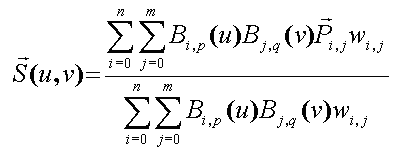In case you were wondering, this is the equation for a rational b-spline surface. If the knot vector used for the basis functions is a non-uniform knot vector, then this is the equation for a non-uniform rational b-spline surface: a NURBS surface! Equation 4 is the equation for a generalized parametric surface. Other common parametric surfaces are just subsets of these surfaces. Specifically, a non-rational, uniform or non-uniform, b-spline surface is one where the weights, wi,j, are all equal to 1. This causes the division to accomplish nothing (and hence we don't have to evaluate the denominator at all). Also, you may have heard of a Bézier surface which is a non-rational b-spline surface with a uniform knot vector that is all zeros followed by all ones. So, for a 3rd degree Bézier surface, the knot vector would be U = {0,0,0,0,1,1,1,1}.

Rational parametric surfaces offer one more nicety that isn't available for non-rational surfaces. Any affine transformation (translation, rotation, scale, shear, and perspective projection) can be applied to the control points of a rational parametric surface and then the surface points generated in the transformed space will be correct. This means that if you have a small number of control points then you can transform the control points and generate a large number of surface points without having to transform all the generated surface points. Using non-rational surfaces, you would at least have to perform the projection transformation of the generated surface points.

Implementing a NURBS Surface Renderer

At this point, we can take Equation 4 and write some code to do a straight forward implementation of this. This would not be too difficult, but there are some optimizations that we can make first so that our implementation will perform better and after all, it's real-time performance that we want. First, let's discuss "tessellation". Tessellation is the process of taking the continuous, mathematical equation of a surface and approximating it with polygons (we'll use triangles). This process can be accomplished in a number of ways with the potential for vastly different visual results.

For simplicity, we're going to use what's called uniform tessellation. Uniform tessellation means we step equally between the minimum and maximum values for the parameters over which the surface is valid. For example, assume that the surface is valid for the ranges u E [0,3] and v E [2,3]. What we can do is divide these into some number of subdivisions and then just loop over these values calculating surface points that will be used as vertices of triangles. If we decide to use 20 subdivisions, we would calculate S(u,v) at u=0, u=0.15, u=0.30, …, u=3 for each v=2, v=2.05, v=2.10, v=2.15, …, v=3. So, we'd end up generating 441 points (21 times 21 because we include the end points) that we could then connect into triangles and render using a 3D API, such as OpenGL* or Direct3D*.

To speed up the calculation of S(u,v), we can calculate Bi,p(u) and Bj,q(v) at the subdivision points and store these in an array. This calculation can be performed once, so that it will not have to be performed in the inner loop of calculating surface points. Instead, a lookup of the pre-computed values and a multiplication is the only task that would be required. If at some point we change the number of subdivisions we want, we can just recalculate the stored arrays of basis functions evaluated at the new subdivisions.

So now that we have a general idea of a way to tessellate a NURBS surface (or any other parametric surface, for that matter), what else do we need? For one, we need a way to generate surface normals so that we can let the 3D API (Direct3D* in the sample code) do lighting calculations for us. How do we generate these? Well, remember those Calculus classes that we all loved? One of the things we learned is that the derivative of a function is the instantaneous slope of the line tangent to the function at the point where the derivative and function are evaluated. By creating two tangent lines (one in the u and one in the v parameter) we can take a cross product and wind up with a surface normal. Simple enough, you say, but what's the derivative of the function S(u,v)?

Well, there are two partial derivatives: one with respect to u andone with respect to v, and they're ugly! Using the chain-rule: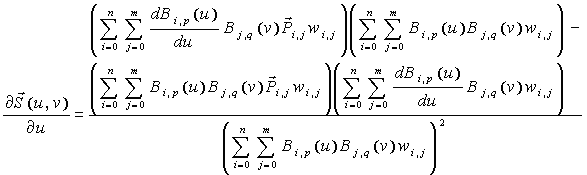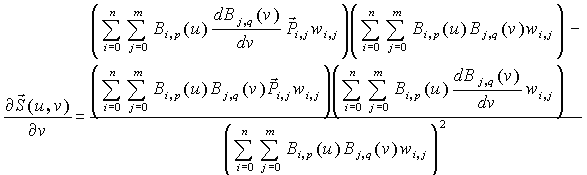And, not only is that ugly, we don't really know how to take the derivatives of Bi,p(u) and Bj,q(v). It's possible to take a derivative of Bi,p(u) (and Bj,q(v) ) from it's definition, but there's an easier way. It's possible to come up with a set of equations for calculating the coefficients of the polynomial equation that Bi,p(u) is equivalent to. Then, taking the derivative of BI,p(u) is as simple as multiplying powers by coefficients and reducing the powers by one (if you recall d(Ax^n + Bx^m)/dx = nAx^(n-1) + mBx^(m-1)). You still have to use Equation 5 to compute the derivatives of S(u,v) but it's really not that bad - you're going to be performing the computation of some of the terms any way, and the ones with the derivatives are calculated the same way as the non-derivative terms. We need to be able to calculate the coefficients of the b-spline basis functions when they're represented as follows: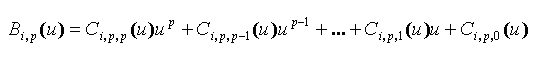Using a lot of paper and a bit of head scratching, I derived the following formulas to compute the coefficients, Ci,p,k(u).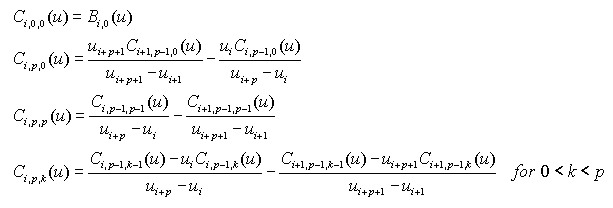This seems complex, but unless the knot vector changes, you don't have to re-compute these coefficients after the first time. Also note that Ci,p,k is only dependent on u for the knot span that u is in not on u itself, so we can just evaluate the Ci,p,k for each knot span and store those values. Now we can write the derivative of Bi,p(u) as: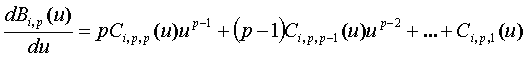Sample Code

At this point we know what we need to know to talk about the sample code you can download and how to implement this fun stuff. First, everything in the sample code is written in C++ and spread across many files of which mainly two are specific to this article: DRGNURBSSurface.h and DRGNURBSSurface.cpp. Actually, you'll also dive into NURBSSample.cpp if you want to play with the surface control points and knot vectors. DRGNURBSSurface.h contains a class definition for a class called CDRGNURBSSurface (for the curious, C is for "class", DRG is for "Developer Relations Group" which is what the group I'm in at Intel used to be called). The methods of this class of interest to us are Init(), ComputeBasisCoefficients(), ComputeCoefficient(), SetTessellations(), EvaluateBasisFunctions(), TessellateSurface(), and TessellateSurfaceSSE().

Going through these in order, Init() is called to initialize a newly created CDRGNURBSSurface object. The function takes a pointer to a CDRGWrapper class that is part of the framework we wrote for getting at the Direct3D* API. Init() also takes two surface degrees, u and v, and the number of control points in the u and v directions. It takes an array of Point4D structures that contain the weighted control points (x, y, z, and w) stored in u-major order (this means that v values are consecutive in the array). It takes two float arrays that contain the u knots and the v knots. Finally, it takes two optional values that specify the number of tessellations in the u and v directions of the surface. Init() does some calculations to determine how many knots are in the knot vectors and then allocates memory to store some of the information needed to render the surface. Finally, Init() makes a local copy of the incoming data (control points and knots) and then calls ComputeBasisCoefficients().

ComputeBasisCoefficients() calls ComputeBasisCoefficient() which uses the formulas from Equation 6 to compute the coefficients of the polynomials formed from the knot vectors and the degrees of the surface. ComputeBasisCoefficient() calls itself recursively due to the definitions in Equation 6. The coefficients are stored in arrays to be used by EvaluateBasisFunctions(). Because the Ci,p,k(u) are only dependent on the knot span that u belongs in, ComputeBasisCoefficient() takes as an argument this knot span (referred to as an "interval" in the code) rather than the actual value of u.

After Init() has called ComputeBasisCoefficients() to do the one-time calculation of the polynomial coefficients, SetTessellations() is called to set the number of u and v tessellations that will be used for rendering the surface. SetTessellations() can be called at any time after initialization to change the fineness of tessellation of the surface. The sample application calls SetTessellations() whenever the plus key (+) or minus key (-) is pressed to increase or decrease the tessellation of the surface. SetTessellations() allocates memory that's dependent on the number of tessellations used for rendering the surface, sets up some triangle indices for rendering the surface, and then calls EvaluateBasisFunctions().

EvaluateBasisFunctions() uses the coefficients computed in ComputeBasisCoefficients() and a technique called "Horner's method" to evaluate the polynomials that are the expanded form of the basis functions. Horner's method says that f = anx^n+an-1x^(n-1)+…+a1^x + a0 can be evaluated using n multiplications and n additions by rewriting as f = a0+x*(a1+x*(a2+…x*(an-1+x*an)…)). If you think you'll be calling EvaluateBasisFunctions() often because your tessellations will be changing, then other optimizations could be made here (e.g. using a technique called "forward differences" to eliminate the multiplications in the inner loop). Additionally, this method could be optimized using the Streaming SIMD Extensions of the Intel Pentium III processor.

At this point, everything is initialized for tessellating a NURBS surface. Now, at each frame that the sample application renders, the Render() method of the CDRGNURBSSurface object is called and in turns calls TessellateSurface() or TessellateSurfaceSSE() depending on whether or not we've told the object to use the Streaming SIMD Extensions of an Intel Pentium III processor.

TessellateSurface() (or TessellateSurfaceSSE()) uses Equation 4 and Equation 5 to compute the surface points and derivatives at the tessellation steps. A cross-product of the derivatives is used to compute the normal to the surface. We don't check for degenerate normals (see the pitfalls section below) so you'll need to modify these routines if degenerate normals become an issue. During the tessellation, a row of triangle vertices is generated. We alternate between putting the vertices in the odd or the even indices of the vertices buffer. Starting with the second row of generated vertices, we call Direct3D* to render a triangle strip using the strip indices generated in SetTessellations(). We alternate between the sets of indices as well due to the winding order of the triangle strip.

Real-Time Optimizations

We already talked about some optimizations that can be done to evaluate NURBS surfaces more quickly. The first, which is used by the sample code, is to use uniform tessellation and pre-evaluate the basis functions and their derivatives at the tessellation points. We also mentioned the possibility of transforming surface control points into projected space and doing our surface tessellation in that space. While this works, lighting can be difficult (or impossible) if you use anything other than directional lights because distance is not preserved in perspective projected space. If you're using light maps in your engine I would highly recommend transforming control points and generating vertices in projected space. You can modify TessellateSurface() to do the divide by homogeneous w and viewport scaling to generate vertices in screen space.

To keep memory requirements minimal, we render the surface by generating two rows of surface points and then passing a triangle strip to the API (Direct3D* in our case). If a surface didn't need to be re-tessellated at every frame, then we could generate all the surface points and store these in an array. Depending on the application, it may still be quicker to tessellate the surface at every frame rather than having to fetch the generated vertices from memory (with corresponding cache misses). You'll need to experiment with your particular application to see what works best.

Aside from the algorithmic optimizations just discussed, we can achieve better performance by using the new Streaming SIMD Extensions supported by Intel's Pentium III processor. These extensions allow us to do mathematical operations on four floating point values at one time (for more information on the Streaming SIMD Extensions of the Intel Pentium III processor, visit http://developer.intel.com/design/pentiumiii/). Since for NURBS surfaces we're dealing with four coordinates (x, y, z, and w) we can do the same operations to all four at once. TessellateSurfaceSSE() uses intrinsic functions provided by the Intel C/C++ Compiler version 4.0 to evaluate all four coordinates of a NURBS surface point at once.

Other optimizations are possible depending on the quality vs. speed tradeoffs acceptable by a particular application. For example, one could choose to generate normals only every other surface point (or less frequently) and then linearly interpolate normals in between.

More Notes on the Sample Code

I should mention a few last things about the sample code contained in the download. The sample requires the Microsoft DirectX 7 SDK to build or run and was written using C++ and built using Microsoft Visual C++ 6.0. If you don't have the Intel C/C++ compiler version 4.0 included with version 4 of the Intel VTune product, you'll need to change a line in DRGNURBSSurface.h. The line reads "#define SUPPORT_PENTIUM_III 1" and should be changed to "#define SUPPORT_PENTIUM_III 0". You can then rebuild everything using the Microsoft compiler (or other C++ compiler) and get to see the code working. You won't be able to enable the tessellation routine that uses the Streaming SIMD Extensions of the Intel Pentium III processor, though.

While running the application, pressing 'H' will bring up a help screen of available keys. Most are self explanatory. One worth mentioning is the 'M' key that causes the display to switch between two different "Objects". The objects are either:

1. A single NURBS surface with 100 control points
2. Nine NURBS surfaces with 16 control points each

You'll notice when viewing the nine surfaces that there are hard creases between the surfaces. This doesn't happen with the single surface. When changing the tessellation level, for the single NURBS surface, there are actually 9 times as many points generated as what the number indicates. This is done to keep a somewhat consistent look between the shapes of the two different "Objects".

I've discussed the math behind parametric surfaces and the basics of rendering them and hopefully made them seem appealing as an alternative to polygonal models. What I haven't addressed are some of the problems that are unique to parametric surfaces and some of the trickier aspects of using parametric surfaces in place of polygonal models.

Some of the more common issues with parametric surfaces are:

1. Texture mapping -- A simple approach to texture mapping a parametric surface is to use the u and v parameter values as texture coordinates (scaled appropriately to the 0 to 1 range). This works fine in some cases (and is what the sample code does), but there may be cases that this won't work for (if the knot vector is very non-uniform, then the texture will be stretched and squashed). To fix this problem, a second parametric surface can be used to generate texture coordinates. This increases overhead substantially, but may be the only solution (and it provides the most flexibility). Many rendering packages allow artists to apply textures to a parametric surface by using a second surface to map the texture coordinates. Keep this in mind as you use parametric surfaces for your applications.
2. Cracking - When two parametric surfaces meet at an edge (or one parametric surface meets a polygonal surface) it's possible for a crack to appear between the surfaces if their degrees of tessellation differ (or it they're just different sizes). This problem can be solved on a per application basis by adding connectivity information to the surfaces. It's not trivial to fix, but it's not impossible.
3. Collision detection - If you're doing collision detection in your application, you have several choices with parametric surfaces:

• Do collision detection on the mesh of control points by treating the mesh as a polygonal mesh - this is approximate and may be too course in some instances.
• Store all the generated triangles and do collision detection on these - while more accurate, it's more memory intensive as well as computationally intensive
• Depending on what types of objects may be colliding, you can solve the parametric surface equations with equations representing the other objects (even lines are difficult, though) and then just plug-and-chug to find collision points
• Use a combination of (a) and (b) by starting with (a) and then refining the surface to triangles to determine an exact hit.
4. Clipping - For surfaces that are partially within the viewing frustum, it can be difficult to clip prior to generating triangles. The problem is that you can't just clip control points because doing so would make the tessellation of the surface difficult to impossible. The easiest solution is to just generate triangles and then clip the triangles - the downside to this is the possibility of generating many more triangles than needed.
5. Back-surface Culling - Aside from clipping, it is also difficult to easily cull back-facing surfaces or portions of surfaces for similar reasons to the clipping problem. For example, a sphere can be defined with one surface but only half of the sphere is ever visible at one time. It would be nice to be able to cull the back-facing portion of the sphere before tessellation, but this is difficult to do.
6. Tessellation - Although a uniform tessellation algorithm is easy to implement and can run fast, in some instances other algorithms may provide better performance/quality. Surfaces that have very curvy areas as well as very flat areas may be better tessellated with a non-uniform tessellation algorithm.
7. Non-local refinement not supported - When refining a surface (i.e. adding detail), you must add control points in complete rows and columns so the control mesh remains a regular grid of points. This causes excessive control points to be added just to add detail in a small, localized region of a surface. Note that this is not an implementation issue, but rather an issue with NURBS surfaces (and other parametric surfaces).
8. Degenerate Normals - Because it's possible to have control points that are at the same location, it's possible for the derivatives of the surface to vanish (i.e. go to zero). This causes the calculation of surface normals to fail. To solve this, it is necessary to look at surrounding points and derivatives if one of the tangents gets too close to zero.

Conclusion

We've covered a lot of information in this article. We've been introduced to parametric curves and surfaces and should have a decent understanding of the concepts behind them. We've learned what's involved in rendering parametric surfaces and can see how the data requirements are smaller than the polygonal models that can be generated. And we should now have an idea how to implement some of the creative types of 3D content we talked about in the introduction.

Given that the field of study of parametric surfaces is enormous, we've only lightly touched the surface (no pun intended) of what's possible. Experimenting with parametric surfaces is exciting. I encourage you to check out the sample code and get a feel for how you can incorporate NURBS surface rendering into your 3D engine today.

Piegl, Les and Tiller, Wayne. The NURBS Book, 2nd Edition, Berlin, Germany: Springer-Verlag, 1996.

Foley, j., van Dam, A., Feiner, S., and Hughes, J. Computer Graphics: Principles and Practice, Reading, MA: Addison-Wesley, 1990.

Dean is a Senior Technical Marketing Engineer with Intel's Developer Relations Division. He is currently researching real-time physics with an emphasis on cloth simulation. He welcomes e-mail regarding NURBS and other parametric surfaces, or anything mathematical and related to real-time 3D graphics. He can be reached at [email protected].

### Latest Jobs

#### Treyarch

Playa Vista, California
6.20.22
Audio Engineer

#### Digital Extremes

6.20.22
Communications Director

6.20.22
Senior Producer

#### Build a Rocket Boy Games

Edinburgh, Scotland
6.20.22
More Jobs